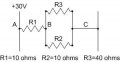# finding current

#### phantomvs

Joined Jan 19, 2016
39I am stuck on this question, that asks me the following :

If R2 is short circuited , the amps in R3 is : ?

Here is what i did :
I redrew circuit without R2. So it becomes a series circuit R1in series with R3
added the resistance. r1 + r3 so 40 +10 = 50
Found the amps so 30v/50 ohm = 0.6 amps
so 0.6 amps times R1 =6 volts on R1 and 30-6 is 24 volts on R3
Since this becomes a series circuit it should be the same current.

What am i doing wrong ?

Last edited:

#### paulktreg

Joined Jun 2, 2008
831
Draw R2 as a straight line (short circuit) and look again.

•phantomvs

#### WBahn

Joined Mar 31, 2012
29,165
Review what it means for something to be short-circuited. Does removing it from the circuit satisfy that meaning?

•phantomvs

#### phantomvs

Joined Jan 19, 2016
39
from what i am reading ,short circuit on R2 automatically makes R3 0 ohms as well.
So pratically removing R3 from circuit.

So 30v/0 ohms is 0 amps

did i get that right ?

#### djsfantasi

Joined Apr 11, 2010
9,080
Automatically makes R3 = 0 Ω?

I don't understand that, do you?

If R2 is shorted, what is the voltage across R2? R3?

#### WBahn

Joined Mar 31, 2012
29,165
from what i am reading ,short circuit on R2 automatically makes R3 0 ohms as well.
So pratically removing R3 from circuit.

So 30v/0 ohms is 0 amps

did i get that right ?
Yes, since shorting R2 also shorts R3. But you need to be careful about saying that it "removes" them from the circuit, since that is basically the mistake you made originally. They are replaced by a wire (the short circuit).

How do you get that 30 V / 0 is 0 amps? What would 30 V / 0.1 Ω be? How about 30 V / 0.000001 Ω? Does that jive with 30 V / 0 Ω being 0 A?

#### WBahn

Joined Mar 31, 2012
29,165
HINT: The key is to ask what the voltage is across R3.

#### phantomvs

Joined Jan 19, 2016
39
The voltage across R3 is 30 volts.
As if there is a short on R2 , then there is a short on R3

So to find amps in R3 would be 30 v / 0 ohms as no resistance just a straight wire .
Voltage at R3 is 30 volts ?

#### WBahn

Joined Mar 31, 2012
29,165
The voltage across R3 is 30 volts.
As if there is a short on R2 , then there is a short on R3

So to find amps in R3 would be 30 v / 0 ohms as no resistance just a straight wire .
Voltage at R3 is 30 volts ?
No. What is the voltage across a short (or across an ideal piece of wire)?

#### phantomvs

Joined Jan 19, 2016
39

#### WBahn

Joined Mar 31, 2012
29,165
Correct. So how much current is flowing in R3?

For extra credit, how much current is flowing in the short?

#### phantomvs

Joined Jan 19, 2016
39
Correct. So how much current is flowing in R3?

0 No current.
If there is no voltage , there is no current .

For extra credit, how much current is flowing in the short?
None as well ?

#### Jony130

Joined Feb 17, 2009
5,457
No current ? Are you sure ? Maybe instead of a short circuit replace R2 with a 0.01Ω resistor.

•phantomvs

#### WBahn

Joined Mar 31, 2012
29,165
None as well ?
As Jony130 already mentioned, consider the case when instead of a dead short (0 Ω) it is just a very low valued resistance such as 1 Ω, or 0.1 Ω, or 0.001 Ω.

Another way to come at it is to ask how much voltage is across R1 and, as a result, how much current is flowing in R1. Where does that current go? How does it get over to the other side (which, presumably, is at 0 V)?

•phantomvs

#### phantomvs

Joined Jan 19, 2016
39
So since it would be only 1 resistor left as the other 2 become short circuited would it be 3 amps .
Voltage on R1 30 volts

If not i am really lost now#### WBahn

Joined Mar 31, 2012
29,165
So, after this 3 A of current leaves R1, where does it go?

#### phantomvs

Joined Jan 19, 2016
39
Well wouldn't it go through R2 /R3

#### WBahn

Joined Mar 31, 2012
29,165
What is the voltage across R2 and R3?

#### phantomvs

Joined Jan 19, 2016
39

#### WBahn

Joined Mar 31, 2012
29,165
This is like pulling teeth!

So what is the current through R2 and R3?